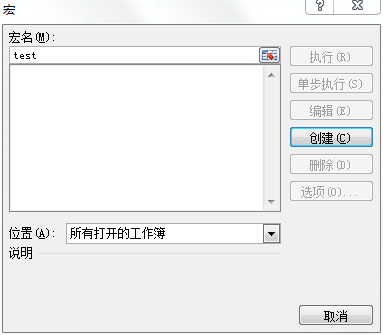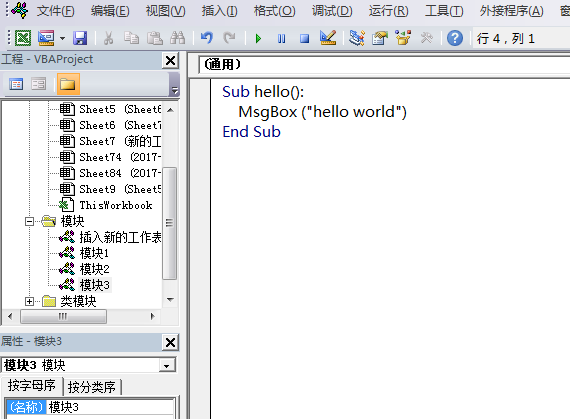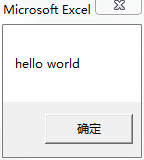## Execl宏教程：从hello world开始入门

Excel宏使用的是vba，基本上就是运行在Excel里面的vb。所以学习vba和学习一门编程语言没有什么区别。所以我们最开始需要学的的就是一些基础语句。为了不让学习显得太枯燥，我们从一个hello world开始。```Sub test()
End Sub```

```Sub test()
MsgBox("hello world")
End Sub
```C++入门：三、函数

Go入门：六、常用标准库

python教程（一）：变量和数据类型

python的基本数据类型有整型，浮点型，字符串，布尔，列表，元组，字典，集合。它们占用内存和定义方式如下表：类型占用内存如何定义整型(int)动态长度>=24字节a = 1浮点型(float)动态长度>=24字节a = 1.0字符串(string)动态长度，>=37字节，增加一个字符加1字节a = ‘str’布尔(boolean)24字节a = True列表(list)动态长度，>=72字节a = []元组(tupe)动态长度，>=56字节a = ()集合(set)动态长度，>=232字节a = set([])占用的内存通过sys.getsizeof()获

C++入门：二、流程控制

matplotlib画柱状图教程

matplotlib画柱状图十分简单，首先需要导入matplotlib中的pyplot，这个就是能够画图的类啦import matplotlib.pyplot as plt画柱状图十分简单，使用bar方法，比如画一个各个班级的人数吧，先准备一些数据，有两组数据，students为人数，这里放到y坐标系中，变量x就放在x坐标系中students = [42,51,30,49]x = [1,2,3,4]好了，现在我们可以直接画出来，调用bar方法，最后再调用show就能画图啦，代码如下plt.bar(x=x, height=students)plt.show()完整代码如下#coding:utf-